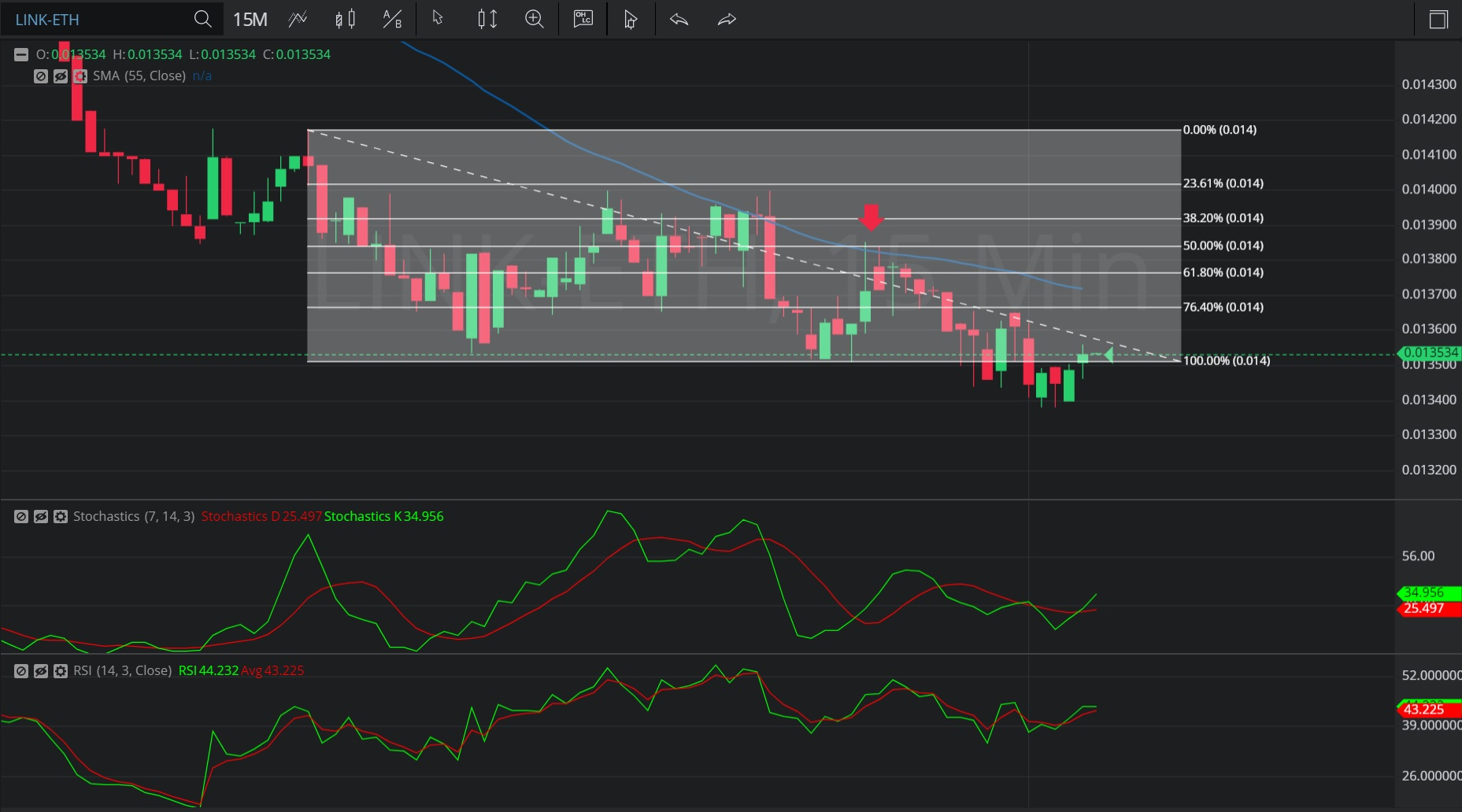Hot Topics

## 6 January 2020 - LINK/ ETH – 15-minuteIn this 15-minute chart of Chainlink/ Ethereum (LINK/ ETH), there is a depreciating range from 0.01418 to 0.01352.

We can observe that the market then appreciated to the 0.013856 level (identified by the red down arrow), just above the 0.01385 area that represents the 50% retracement of the depreciating range.

The 0.013856 level was also just above the 55-bar Simple Moving Average, which was indicating around the 0.013831 level.

We can observe that the market then depreciated to the 0.013382 area.

Using RSI, we can observe that the slope of RSI (14) became negative a few bars after the market traded as high as the 0.013856 level, and then RSI Average (3) bearishly moved above RSI (14). We can also observe the slope of RSI (14) turned positive after the market traded as low as the 0.013382 level, suggesting a price reversal could be taking place. This was confirmed after RSI (14) moved back above RSI Average (3).

Using Slow Stochastics, we can observe that the slope of Stochastics K turned negative after the market traded as high as the 0.013856 level, and thereafter Stochastics D bearishly moved above Stochastics K.  We can also observe that Stochastics K has moved back above Stochastics D, suggesting price depreciation may have ended. The slopes of both Stochastics K and Stochastics D are positive, supporting this likelihood.

Stay on top of the cryptocurrency market with CryptoDaily’s trading charts!

https://charts.cryptodaily.net/